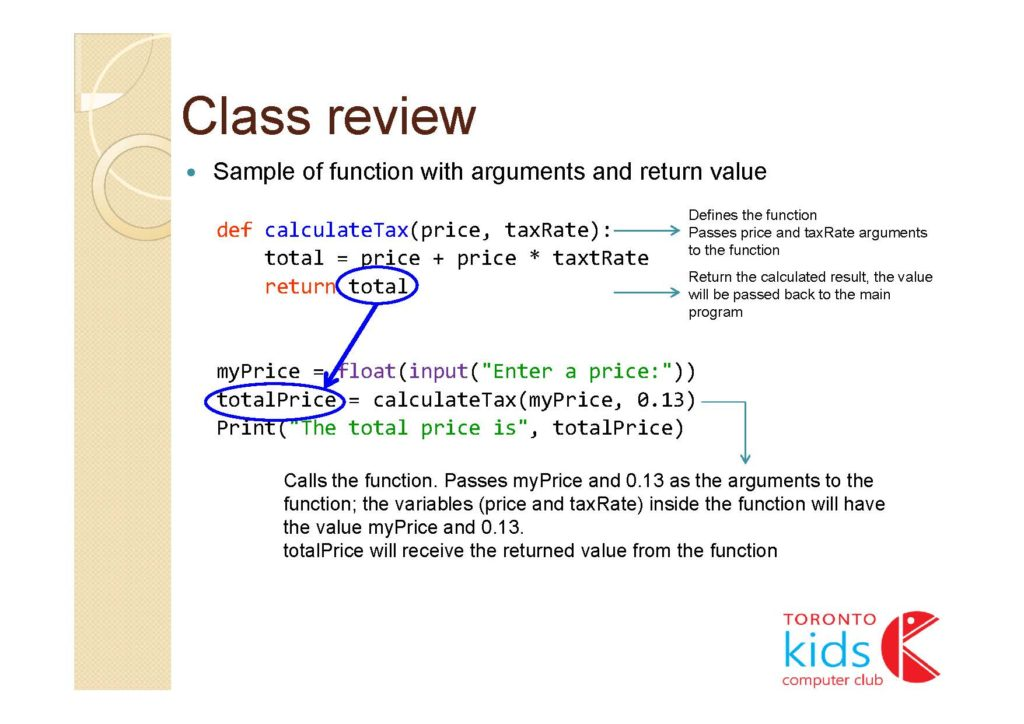TORONTO KIDS COMPUTER CLUB | Aurora Tuesday 18:30 Python Homework 20.06.16.
17711

# Aurora Tuesday 18:30 Python Homework 20.06.16.

## 18 Jun Aurora Tuesday 18:30 Python Homework 20.06.16.

Question:

The factorial function (symbol: !) says to multiply all whole numbers from our chosen number down to 1.

Examples:

• 4! = 4 × 3 × 2 × 1 = 24
• 7! = 7 × 6 × 5 × 4 × 3 × 2 × 1 = 5040
• 1! = 1

Factorial is not defined for negative numbers, and the factorial of zero is one, 0! = 1

Write a function to find the factorial of a number. The function should take one number as an argument and print the factorial of that number

```Sample Input:
5

Sample Output:
120```
```Hint:
The factorial of 5 is 1 * 2 * 3 * 4 * 5 = 120 and also use loops inside the function```

Class review: